Search by Topic

Resources tagged with Algorithms similar to Route to Root:

Filter by: Content type:
Age range:
Challenge level:

There are 24 results

Broad Topics > Decision Mathematics and Combinatorics > AlgorithmsRoute to Root

Age 16 to 18 Challenge Level:

A sequence of numbers x1, x2, x3, ... starts with x1 = 2, and, if you know any term xn, you can find the next term xn+1 using the formula: xn+1 = (xn + 3/xn)/2 . Calculate the first six terms of this. . . .Peaches in General

Age 14 to 16 Challenge Level:

It's like 'Peaches Today, Peaches Tomorrow' but interestingly generalized.Unusual Long Division - Square Roots Before Calculators

Age 14 to 16 Challenge Level:

However did we manage before calculators? Is there an efficient way to do a square root if you have to do the work yourself?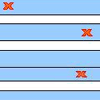Stretching Fractions

Age 14 to 16 Challenge Level:

Imagine a strip with a mark somewhere along it. Fold it in the middle so that the bottom reaches back to the top. Stetch it out to match the original length. Now where's the mark?Happy Birthday

Age 16 to 18 Challenge Level:

Can you interpret this algorithm to determine the day on which you were born?Jluuis or Even Asutguus?

Age 16 to 18 Challenge Level:

Sixth challenge cipher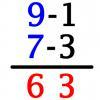Vedic Sutra - All from 9 and Last from 10

Age 14 to 16 Challenge Level:

Vedic Sutra is one of many ancient Indian sutras which involves a cross subtraction method. Can you give a good explanation of WHY it works?Semicircle

Age 16 to 18 Challenge Level:

Fourth challenge cipher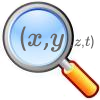Stage 5 Cipher Challenge

Age 16 to 18 Challenge Level:

Can you crack these very difficult challenge ciphers? How might you systematise the cracking of unknown ciphers?Zeller's Birthday

Age 14 to 16 Challenge Level:

What day of the week were you born on? Do you know? Here's a way to find out.A Fine Thing?

Age 16 to 18 Challenge Level:

Second challenge cipherVital?

Age 16 to 18 Challenge Level:

Third challenge cipherUp a Semitone?

Age 16 to 18 Challenge Level:

Fifth challenge cipherIp?

Age 16 to 18 Challenge Level:

Seventh challenge cipher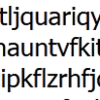Probably a Code?

Age 16 to 18 Challenge Level:

Is the regularity shown in this encoded message noise or structure?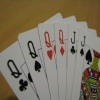Sorted

Age 14 to 18 Challenge Level:

How can you quickly sort a suit of cards in order from Ace to King?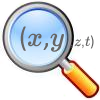The Best Square

Age 14 to 18 Challenge Level:

How would you judge a competition to draw a freehand square?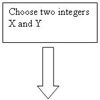Procedure Solver

Age 16 to 18 Challenge Level:

Can you think like a computer and work out what this flow diagram does?Divided Differences

Age 16 to 18

When in 1821 Charles Babbage invented the `Difference Engine' it was intended to take over the work of making mathematical tables by the techniques described in this article.Geomlab

Age 11 to 18 Challenge Level:

A geometry lab crafted in a functional programming language. Ported to Flash from the original java at web.comlab.ox.ac.uk/geomlabTriangle Incircle Iteration

Age 14 to 16 Challenge Level:

Keep constructing triangles in the incircle of the previous triangle. What happens?An Introduction to Computer Programming and Mathematics

Age 16 to 18

This article explains the concepts involved in scientific mathematical computing. It will be very useful and interesting to anyone interested in computer programming or mathematics.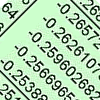Odd One Out

Age 16 to 18 Short Challenge Level:

In this short problem, can you deduce the likely location of the odd ones out in six sets of random numbers?Tournament Scheduling

Age 11 to 16

Scheduling games is a little more challenging than one might desire. Here are some tournament formats that sport schedulers use.